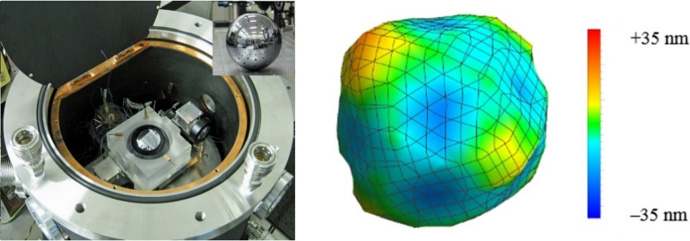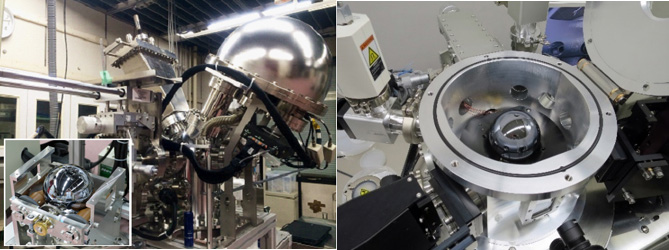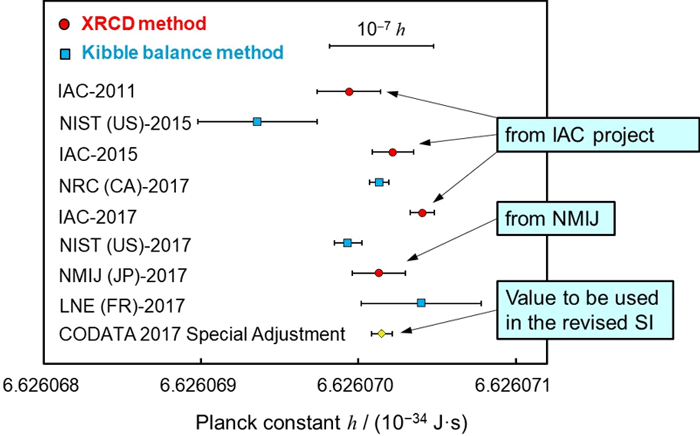Update(MM/DD/YYYY):06/25/2019

# Determination of the Planck constant that is to be used as the new definition of the unit of mass, the kilogram

– The Planck constant was measured with world’s highest-level precision –

## Points

• The Planck constant has been measured at a level of precision better than the long-term mass stability of the International Prototype of the Kilogram.
• Contributed to determining the Planck constant, which is newly used for the redefinition of the kilogram, by the Committee on Data of the International Science Council.
• Historic outcome contributes to the first redefinition of the kilogram in about 130 years.

## Summary

Kenichi Fujii (Prime Senior Researcher), the Research Institute for Engineering Measurement (Director: Toshiyuki Takatsuji), the National Institute of Advanced Industrial Science and Technology (AIST; President: Ryoji Chubachi), Naoki Kuramoto (Senior Researcher) and Shigeki Mizushima (Senior Researcher), Mass Standards Group of the same Institute, Lulu Zhang (Senior Researcher), Surface and Nano Analysis Research Group, the Research Institute for Material and Chemical Measurement (Director: Akiko Takatsu), AIST and others have measured the Planck constant, one of the fundamental physical constants, with the world’s highest level of precision by making ultra-precise measurements of the shape of a single-crystal silicon sphere, thereby making a significant contribution to the redefinition of the kilogram.

The kilogram has been exactly defined as the mass of the International Prototype of the Kilogram (IPK), a weight that is the only one of its kind in the entire world. The mass of IPK, however, has been found to fluctuate over a long term, partly because of contamination of its surface. Because of this, research has been conducted around the world to determine a fundamental physical constant at a level of precision greater than the long-term stability of the mass of IPK to revise the definition of the kilogram based on a universal fundamental physical constant. As a result, it will be debated in 2018 whether to change the definition of the kilogram that is based on the Planck constant.

The AIST researchers recently measured the Planck constant at the world’s highest level of precision by measuring the shape of a single-crystal silicon sphere, approximately 94 mm in diameter, with sub-nanometer precision by using an ultra-high-precision laser interferometer and a surface analysis system. The Committee on Data of the International Science Council (CODATA) has determined the value of the Planck constant to be used for the new definition of the kilogram based on the high-precision Planck constant measurement results of AIST and a number of research institutes outside Japan. This is the first time Japan has been directly involved in defining a basic unit of the International System of Units (SI). It is a historical outcome in that it contributes to the first revision of the kilogram’s definition in about 130 years.

Details about the setting of the Planck constant by CODATA were published as an accepted manuscript in Metrologia, a journal of the Institute of Physics in Great Britain, on October 21, 2017 local time.A laser interferometer developed by AIST that measures the shape of a single-crystal silicon sphere with high precision (left) and three-dimensional image of the sphere’s shape, showing deviations from the mean diameter (right)

## Social Background of Research

Measurement is not only fundamental to science but also has a significant impact on community life in the form of product trade and legal regulations, so a highly reliable global system of units is indispensable. SI is the most widely used system of units today. It benefits all aspects of modern society, from day-to-day life to leading-edge science.

The kilogram (the unit of mass) and meter (the unit of length) are important basic units of SI. The first General Conference on Weights and Measures (CGPM) defined the kilogram as the mass of the platinum-iridium IPK and the meter as the length of the “International Prototype of the Meter.” The definitions of units have continued to evolve since then, incorporating the latest science and technology, to enhance the reliability of measurement. Following advances in laser technology, the definition of the meter was revised in 1983. That definition is based on a universal fundamental physical constant: the speed of light in a vacuum. This made it possible to set the standard of length at a level of precision 1000 times greater than the International Prototype of the Meter.

The definition of the kilogram, on the other hand, has not changed in about 130 years. Although IPK is kept under strict control by the International Bureau of Weights and Measures (BIPM), the stability of its mass is estimated to be approximately 50 µg over about a century, partly because of surface contamination. This range of fluctuation is just 50 billionths (5 × 10−8) of 1 kg, but that much fluctuation is not negligible in the latest measuring technology.

Therefore, the national metrology institutes (NMIs) of various countries began attempting to measure one of the approximately 200 universal fundamental physical constants precisely enough to overcome that 5 × 10−8 fluctuation and to use that value as the basis for defining the kilogram. As a result, it was decided at the 24th CGPM in 2011 to abolish IPK in the future and redefine the kilogram to read as follows:

“The magnitude of the kilogram will be set by fixing the numerical value of the Planck constant to be equal to exactly 6.626 07X XX × 10−34 J s.”

The Planck constant is one of the most important fundamental physical constants in quantum theory and is related to the mass of an electron. For that reason, the current 1 kg can be represented by the Planck constant. In addition, X XX is to be decided based on reliable measurements to be taken at the time the definition is revised.

It was decided at the 25th CGPM in 2014 that NMIs of various countries would prepare so that the redefinition of the kilogram could be discussed at the 26th CGPM in 2018. In response, NMIs were asked to announce by July 1, 2017 the results of the measurements they would take to help decide the value of the Planck constant as the basis for the new kilogram.

## History of Research

There are two methods of measuring the Planck constant. One is called the Kibble balance method and the other is the X-ray crystal density (XRCD) method. AIST began taking precise measurements of the Planck constant about 40 years ago using the XRCD method. This method measures the density, molar mass, and lattice constant of a silicon single crystal and count the number of atoms in the silicon single crystal to measure the Avogadro constant. There is a strict numerical relation between the Planck and Avogadro constants, and so the Planck constant can be calculated from measurements of the Avogadro constant at about the same level of precision. In the natural world, silicon has three stable isotopes (28Si, 29Si, and 30Si), so determination of the molar mass requires isotopic ratio measurement. This was a bottleneck that limited the precision of the Avogadro constant measurement to 3 × 10−7. For that reason, AIST launched in the International Avogadro Coordination (IAC) Project, which produced a 28Si single crystal enriched to 99.99 % 28Si purity. In 2011, IAC used this 28Si single crystal to measure the Planck constant at 3 × 10−8 (30 billionths) precision, which at the time was the world’s highest level of precision (AIST Research Results, February 27, 2012). This level of precision exceeds the long-term stability of IPK, but the Planck constant that it derived did not conform to that of the National Institute of Standards and Technology (NIST) of the U.S.A., which used the Kibble balance method. For that reason, the 24th CGPM in 2011 only decided to adopt a policy of transitioning to a new definition of the kilogram based on the Planck constant sometime in the future and the definition itself was not revised at this time.

## Details of Research

This measurement of the Planck constant used a sphere polished from the 28Si single crystal produced by the IAC Project. The sphere’s mass and diameter are approximately 1 kg and 94 mm, respectively; the mass and volume were measured precisely to determine the density. A laser interferometer developed by AIST was used to measure the volume. The diameter of the sphere was measured from about 2,000 directions, enabling the sphere’s volume to be determined at a precision of 2 × 10−8. The diameter was measured with a precision of 0.6 nm, which is approximately equivalent to the atomic distance (lattice constant) of silicon. This world’s most precise measurement of diameter was achieved through a combination of laser wavelength precision control technology and precise sphere temperature measurement technology (with precision of 6/10 000 °C) which were developed by AIST. The mass of the silicon sphere was measured by comparing it to the National Prototype of the Kilogram of Japan (NPK), Japan’s mass standard, using a vacuum balance capable of comparing masses with super-high precision.

The surface of the silicon sphere is covered with layers of a few nanometer thick, consisting of such matter as oxide. To count the silicon atoms and determine the Planck constant, it is necessary to determine the mass and volume of the part consisting only of silicon atoms (the silicon core). For this purpose, a new spherical surface analysis system using X-ray photoelectron spectroscopy and spectroscopic ellipsometry was developed (Fig. 1). Both devices have a mechanism to rotate the silicon sphere, so both can analyze the entire surface of the sphere. The system determined the composition of the surface layer of the sphere and measured the thickness of the surface layer with precision of 0.1 nm. The results of this surface layer analysis were used to correct the measured values of the silicon sphere’s mass and volume and determine the mass and volume of the silicon core.Fig. 1 X-ray photoelectron spectroscopy system (left), and spectroscopic ellipsometer (right), both developed by AIST Together, the devices can determine the composition of the sphere’s surface layer and measure the thickness of the surface layer with the world’s highest precision of 0.1 nm. Both devices have a mechanism to rotate the sphere, so both can analyze the entire surface of the sphere.

The mass and volume of the silicon core measured in this manner were combined with the lattice constant and molar mass measured previously by the IAC Project to determine the Planck constant at the world’s highest level of precision, 2.4 × 10−8 (24 billionths). That precision is equivalent to 24 µg at 1 kg level, surpassing the mass stability of IPK, which is 50 µg.

Figure 2 shows values of the Planck constant reported by various nations’ NMIs as of July 1, 2017. The value shown after NMIJ (JP)-2017 is the one determined by AIST. It is a close match to those values measured by the IAC Project (IAC-2011, IAC-2015, and IAC-2017). It is also a close match to values that NIST of the U.S.A., the National Research Council (NRC) of Canada, and le Laboratoire national de métrologie et d’essais (LNE) of France measured by the Kibble balance method (NIST (US)-2015, NIST (US)-2017, NRC (CA)-2017, and LNE (FR)-2017).

CODATA determined an adjusted value for the Planck constant (CODATA-2017; 6.626 070 150(69) × 10−34 J s) based on these eight high-precision measurements (numerical values in parentheses indicate the standard uncertainty of the last digit). The relative standard uncertainty of CODATA-2017 is 1.0 × 10−8 (10 billionths). That precision is equivalent to 10 µg per kg, far surpassing the 50 µg mass stability of IPK. At the 26th CGPM held in November 2018, the transition to a new kilogram definition based on the CODATA-2017 definition with zero uncertainty will be discussed.Fig. 2 The adjusted value for the Planck constant as determined by CODATA (CODATA-2017) and the eight measurement results used to determine the value At the 26th CGPM in November 2018, the transition to a new kilogram definition based on this adjusted value with zero uncertainty will be discussed.

Of the eight data points used to set CODATA-2017, AIST contributed to the measurement of four, and of those, one was measured by AIST as described above. It was mainly North America and Europe that developed most of the system of units that underlies science and technology, but now, for the first time in the long history of metrology, a country from outside those areas has played a decisive role in revising the international system of units. Moreover, AIST’s measurement result is expected to contribute greatly to the first redefinition of the kilogram in about 130 years.

## Future Plans

If a decision is made at the 26th CGPM in November 2018 to revise the definition based on the Planck constant, the plan is to put the new definition into effect on the World Metrology Day (May 20), 2019.

In light of the expectation that the definition will be revised, AIST has begun research and development for high-precision measurement of very small mass in the sub-milligram and microgram range, for which there is a strong need in fields like new pharmaceutical development (drug discovery) and environmental measures of microparticles, etc. based on the Planck constant. Presently, high-precision measurement of mass requires weights whose masses are assigned in respect to IPK, but weights are not practical for very small mass because of problems like long-term mass stability. AIST, therefore, aims to develop new measuring technologies for very small mass based on the Planck constant.

▲ ページトップへ